## Fun with Chi-Squared

As mentioned before, the Chi-Squared inverse cumulative distribution is used when calculating confidence intervals in allantools.

When comparing computed confidence intervals against Stable32, there seems to be some systematic offsets, which I wanted to investigate.

While allantools uses scipy.stats.chi2.ppf(), It turns out that Stable32 uses an iterative method for EDF<=100, and an approximation from Abramowitz and Stegun: Handbook of Mathematical Functions for EDF>100. This 1964 book seems to be available online from many sites. The approximation (for large EDF) for the inverse chi-squared cumulative distribution is equations 26.4.17 (p410 in the PDF) (here nu is the equivalent degrees of freedom)

Where xp is from the inverse of the Normal cumulative distribution, computed via the approximation 26.2.22 (p404 in the PDF)

It also looks like the Stable32 source contains a misprint, where the a1 coefficient of 26.2.22 is used with two digits in the wrong order! (I did promise 'fun' in the title of this post!)

``a1=0.27601   # typo in the Stable32 source code!? should be 0.27061``

If we plot both scipy.stats.chi2.ppf() and the Stable32 implementation for various EDF and probabilities we get the following:

The leftmost figure shows Chi-Squared values (divided by EDF, to overlap all curves). There's not much difference between scipy and Stable32 visible at this scale. The middle figure shows the difference between the two algorithms. This shows very clearly the small (<100) EDF-region where the iterative algorithm in Stable32 produces a Chi-squared value in agreement with scipy to better than 4 digits (see the noisy traces around zero). This plot also reveals the shortcomings of the Abramovitz & Stegun approximation, together with the source-code misprint. The errors are largest, up to almost 1e-3 (in chi-squared/EDF) for EDF=101, and decrease with higher EDF. The rightmost figure shows what impact this has on computed confidence intervals - shown as relative errors. The confidence interval is proportional to sqrt(EDF/chi-squared). The dashed lines show the probabilities corresponding to 1-sigma confidence intervals, where we usually sample this function.

For 1-sigma confidence intervals we can now compare computed results with Stable32 and allantools to the comparison above of scipy and Abramovitz&Stegun + misprint.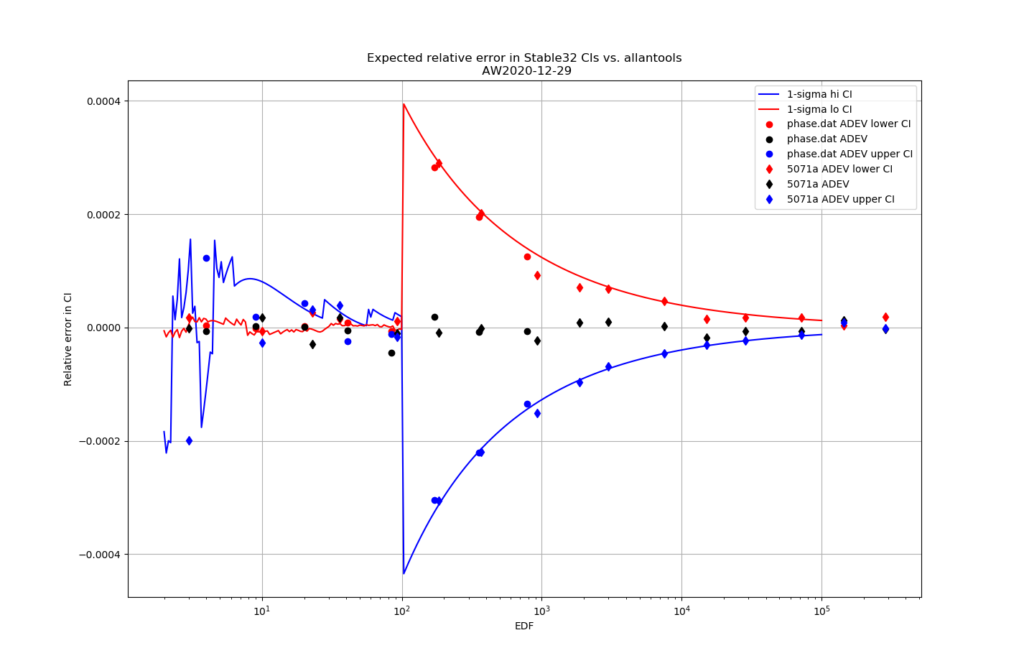Predicted and computed relative error for confidence intervals computed with Stable32 vs. allantools. The lower bound is shown in red, and the upper bound in blue. The relative error of the computed ADEV is shown as black symbols, for reference. The symbols are from computed deviations and confidence intervals for two test-datasets in allantools: phase.dat and a 5071a-dataset.

Are we having fun yet!? The comparison above between the two methods to compute the inverse chi-squared cumulative distribution seems to predict how Stable32 confidence intervals differ from allantools confidence intervals quite well! Note that Stable32 results are copy/pasted from the GUI with 5 significant digits, so some noise at the 1e-4 level is to be expected. Note that the relative error has a different sign depending on if we look at the lower or upper bound, and also depending on if we use the EDF<=100 iterative algorithm or the EDF>100 Abramovitz&Stegun+misprint approximation. For large EDF the upper bound (blue) of Stable32 is a bit too low, while the lower bound (red) is a bit too high.

Finally, the chi-squared figure and the expected confidence interval relative error figure, if we fix the misprint in the a1 coefficient: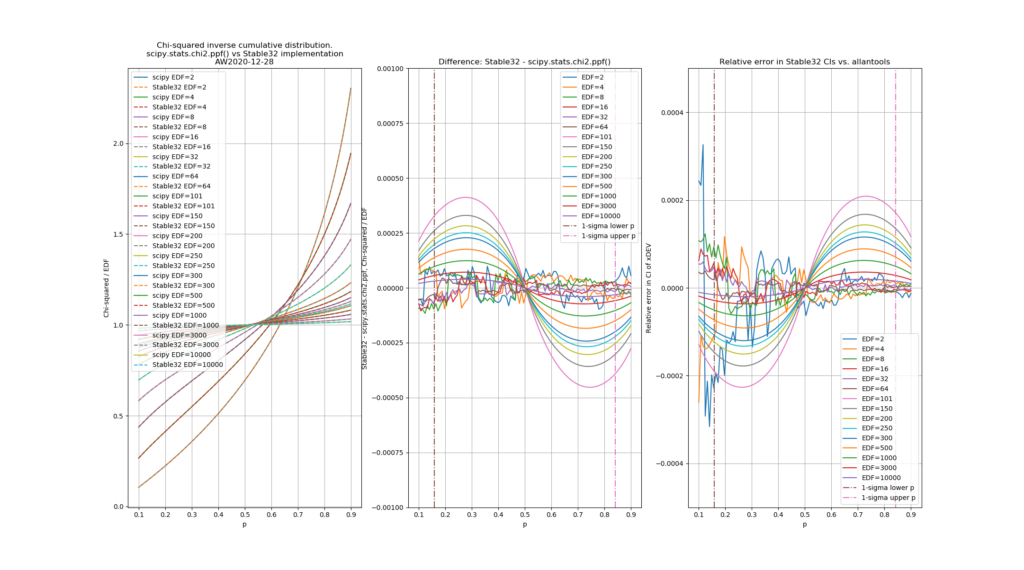Note with the a1-coefficient misprint fixed the approximation is exact at p=0.5, and there is no discontinuity in the difference-curves.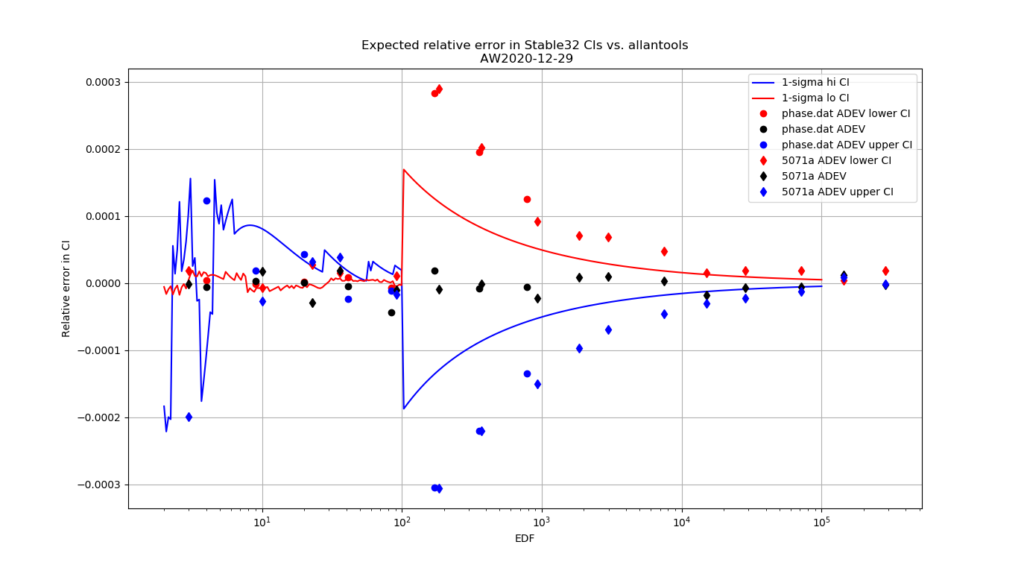If the a1-coefficient misprint would be fixed in Stable32, we could expect the EDF>100 confidence interval relative errors between Stable32 and allantools to be roughly halved. The data-symbols are the same as in the figure above - agreement between the lines and symbols is not expected here.

Python code for producing these figures is available: chi2_stable32.py

The approximations used by Stable32 are not (yet?) included in allantools.

## Modified Total Deviation in allantools

I wrote a first rough (and very slow!) implementation of Modified Total Deviation mtotdev() for allantools.

mtotdev() combines the features of modified Allan deviation mdev() (being able to distinguish between white and flicker phase modulation) and Total deviation totdev() (better confidence intervals at large tau).

Here are some results. The Time Total Deviation ttotdev() follows trivially from this work also, since it is only a scaled version of mtotdev(). I used the "NBS14" 1000-point frequency dataset and compared my results against Stable32 and those in NIST SP 1065 (Table 31, page 108).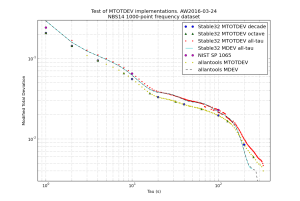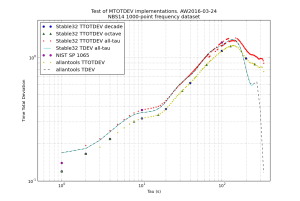The figures show mtotdev() and ttotdev() from Stable32 runs and allantools. I've also added mdev() and tdev() traces to compare against. At first sight this looks strange, but mtotdev() is a biased estimator, and Stable32 applies a bias correction which explains the results.

Somewhat surprisingly Stable32 applies a bias-correction only when run in the "all-tau" mode. These numbers agree with those from the NIST SP 1065 table. For this dataset Stable32 is undecided on what power-law the dataset follows at large tau, which results in deviations that jump up and down (because a different bias-correction is applied at different tau, red datapoints).

When Stable32 is run in "octave-tau" or "decade-tau" mode no bias correction is applied. These numbers agree with the ones from allantools.mtotdev().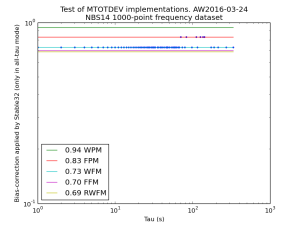This figure shows the ratio of variances between allantools.mtotdev() (no bias correction) and a Stable32 all-tau run (bias correction applied). The lines correspond to the bias-correction values for different noise processes.

There also seems to be a misprint in NIST SP 1065 equation (28) page 26, where it says ttotdev() is scaled by tau-cubed (when tau-squared is correct). Who reads these things anyway - not many it seems 😉